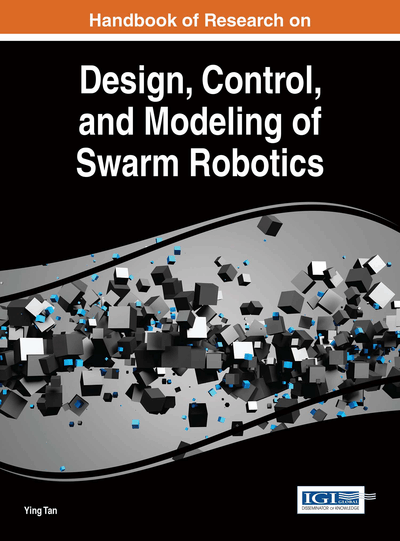# Macroscopic Group Robots Inspired By “Brownian Motion”

Teturo Itami (Robotics Industry Development Council, Japan)
Copyright: © 2016 |Pages: 35
DOI: 10.4018/978-1-4666-9572-6.ch018
OnDemand PDF Download:
Available
\$37.50
No Current Special Offers

## Abstract

The sections contained in this chapter, Macroscopic Group robots inspired by “Brownian motion”, examine the basic concepts and the methods to develop systems of new type of group robots. We take robots especially with neither external sensors nor apparatuses for mutual communication. In microscopic physics we have a phenomena of Brownian motion. We note that in Brownian motion pollen particles can be motivated by collision with surrounding molecules although each molecule has neither sensor nor mutual communication. By setting temperature gradient surrounding the pollen particle, controlling its motion is possible. So when we note correspondence between molecules and robots, we will obtain a transportation system of macroscopic objects that correspond to pollen particles in Brownian motion. We use a potential force signal that corresponds to temperature in Brownian motion. To perform the tasks, we show and solve various challenging problems in fundamental formulae, simulation scheme, and control method in these areas.
Chapter Preview
Top

## Introduction

A new type of group robots in macroscopic world is proposed in the chapter. Our objective is controlling a motion of an object by many robots that repetitively collide to the object. In our final goal we equip our robots with neither external sensor nor apparatuses for mutual communication. The way in which Brownian motion works in microscopic physics makes the group robots act to transport an object. To carry out our objectives, we need an appropriate control scheme that requires mathematical model of the systems. A word “macroscopic” means that our systems are subject to classical Newton mechanics. We take robots especially with neither external sensors nor apparatuses for mutual communication. In conventional systems of group robots we need various external sensors mainly for collision free goal. Apparatuses for mutual communication are also necessary to achieve cooperative motion. The equipment and increase of communication paths make systems complex and result in high cost. The mechanism of Brownian motion helps us to develop the systems of group robots that cannot see, hear, smell their external world. In microscopic physics we have the phenomena of Brownian motion. Pollen particles floating on liquids fluctuate by collision with liquid molecules. We note that in Brownian motion the pollen particles can be motivated by collision with the surrounding molecules although each molecule has neither external sensor nor mutual communication. Among the molecules around the pollen particle energy proportional to temperature value is distributed. By setting a temperature gradient surrounding the pollen particle, controlling its motion is possible. When we note correspondence between the molecules and the robots, we will obtain a transportation system of macroscopic objects that correspond to the pollen particles in Brownian motion. But we have serious problems that our systems certainly differ from Brownian motion. Firstly, the temperature gradient is too small to work as force of transportation. Also the established stochastic Langevin’s equation for Brownian motion is not appropriate to our systems. In the chapter we make an external potential field play a role of the temperature gradient. Each robot moves as if it were a physical ball under the potential. Repetitive collisions of robots force the object follow some required transportation path. The tracking is controlled by a form of the potential function. Regarding a governing equation of the system, continuum mechanics is shown to efficiently describe classical Newton mechanics of the robots. After analysis of collision process of robots and an object, we show numerical simulation and tracking control in transportation systems. Validity is examined by comparing those with numerical simulation that are given by directly solving many-body Newton equations. The sections in this chapter show fundamental formulae, simulation results, and control method in these areas.

After we show new transportation systems inspired by Brownian motion, how to efficiently describe our systems is examined in detail. According to the analysis of collision process, we do simulation studies. Emphasis is on comparison of continuum mechanical calculation with those by direct simulation using Newton equations. We control macroscopic Brownian motion by group robots. To make an object track a required path, feedforward control input is found using our model of continuum mechanical description. In the final part of the chapter, an improvement of the continuum model and an experiment on control by potential force are given as future directions of research.

## Key Terms in this Chapter

Potential Force: Mechanical force that is calculated as negative gradient of potential function. Usually the potential function is a function of coordinate of mass point and time.

Feedforward Control: A control scheme of a system where inputs are designed by a system model and no measurement of outputs is necessary.

Distribution Function: A density function that gives, in a multiplied form of coordinate and momentum interval, probability of classical mass points being between some coordinate and momentum interval.

Group Robotics: Robotics technology that features cooperation of many collected robots.

Temperature Parameter: One of two parameters that characterize one body distribution function in its equilibrium form. Another parameter is only a proportionality constant of the one body distribution function.

Relaxation Time: A time parameter in that duration distribution function approaches to its equilibrium form.

Liouville Equation: Classical mechanical equation that gives a rule of time development of distribution function.

Energy Dissipation: A physical quantity that total energy of classical mass point loses due to friction.

Brownian Motion: Displacement fluctuation of pollen particles due to collision of surrounding liquid molecules.

One Body Distribution Function: A probability density function for one classical mass point. This is obtained by integrating out a distribution function by coordinates and momenta of other mass points.

Continuum Mechanics: A physical theory to explain characteristics of liquid or solid.

## Complete Chapter List

Search this Book:
Reset